# Basic Geometry : How to find the length of the diameter

## Example Questions

1 2 3 4 5 7 Next →

### Example Question #61 : How To Find The Length Of The Diameter

The area of a circle is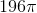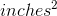. What is the diameter?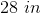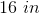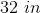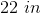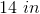Explanation:

To find the diameter, we must first find the radius using the formula to find a circle's area.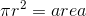We have the area, so we have to work backwards.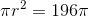Divide both sides by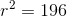Take the square root of both sides.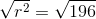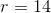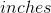Now, since you have the radius, you have to double it to get the diameter.

diameter = 28 inches

### Example Question #62 : How To Find The Length Of The Diameter

The area of a circle is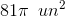. What is the diameter?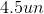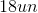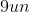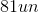Explanation:

The area of a circle is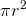, which means that in this circle, the radius squared is 81. The square root of 81 is 9, which means that the radius is 9. The diameter is just twice the length of the radius, so in this case 9 times 2, which is 18.

### Example Question #63 : How To Find The Length Of The Diameter

The area of a circle is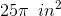. What is the length of its diameter?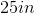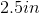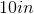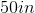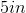Explanation:

The area of a circle is. In this case,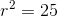. Taking the square root, the radius has a length of 5. To find the diameter, multiply by 2. Two times five is 10, so the diameter has a length of 10.

### Example Question #64 : How To Find The Length Of The Diameter

True or false: A circle with radius 20 has diameter 10.

True

False

False

Explanation:

The diameter of a circle is twice its radius (not the other way around), so a circle with radius 20 has diameter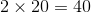.

### Example Question #65 : How To Find The Length Of The Diameter

True or false: A circle with radius 500 centimeters has diameter one meter.

False

True

False

Explanation:

The diameter of a circle is twice that of the radius, so the diameter of a circle with radius 500 centimeters is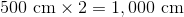. However, one meter is equal to 100 centimeters, so the statement is false.

### Example Question #66 : How To Find The Length Of The Diameter

Use 3.14 for Pi in the following question.

If the circumference of a circle is 25.12cm, what is the measure of its diameter?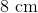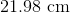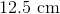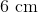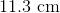Explanation:

First we need to know that the formula for circumference of a circle is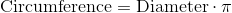.

Since we know the circumference, we can plug it into our equation, along with pi, and solve for diameter.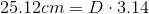To solve for D (diameter) we must divide both sides by pi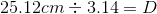when we divide 25.12 by 3.14 we get 8, so our final answer is 8cm.

### Example Question #67 : How To Find The Length Of The Diameter

A circular pizza has an area ofsquare inches. What is its diameter in inches?Explanation:

The area of a circle with a given radiusis. Since the area of the circular pizza is given assquare inches, we can solve for its radius as follows: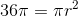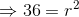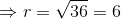Now that we know that the radius of the pizza isinches, we can now deduce its diameter knowing that the diameter of a circle is equal to twice its radius. Hence, the diameter of this pizza is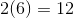inches.

1 2 3 4 5 7 Next →